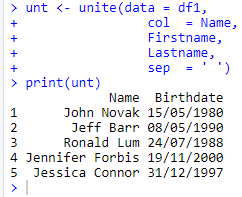top of pageSearch

# Data Manipulation using Tidyr : Part 2

In this blog, you will learn how to perform data manipulation using the tidyr R package. The tidyr package has been designed for the specific task of taking our data in its messy format and getting it into a tidy structure, conductive for data analysis. We will discuss the following functions:

• separate

• unite

• pivot_wider

• pivot_longer

# What is Tidy data?

There are many ways to represent the same underlying data in a data set. It is important, as a data analyst, to be able to structure your data in a way that is efficient for data analysis. If the data set is not the optimal format for analysis, we must reshape, or ‘tidy’ it.

There are three rules which make a data set tidy:

1. Each variable forms a column

2. Each observation forms a row

3. Each value must have its own cell

# Required R package

First, you need to install the tidyr package and load the tidyr library then after you can able to perform the following data manipulation functions.

```install.packages('tidyr')
library(tidyr)```

# Demo Dataset

```df1 <- data.frame(Firstname = c('John', 'Jeff', 'Ronald', 'Jennifer', 'Jessica'),
Lastname = c('Novak', 'Barr', 'Lum', 'Forbis', 'Connor'),
Birthdate = c('15/05/1980', '08/05/1990', '24/07/1988', '19/11/2000', '31/12/1997'))
print(df1)```

Output:# 1. The Separate Function

Sometimes, a column contains two or more variables. In the demo data set, the Birthday column contains the variable Date, Month, and Year. If we need to work with these three as a separate variable then we can use the separate() function. This function pulls apart one column into multiple columns, by splitting wherever a separator character appears.

```sept <- separate(data = df1,
col  = Birthdate,
into = c('Date', 'Month', 'Year'),
sep = '/')
print(sept)```

Output:# 2. The Unite Function

The unite() function combines multiple columns into a single column. It is the inverse of the separate() function. In the demo data set, if we want to combine the variable ‘Firstname’ and ‘Lastname’ in Name column then we can use unite() function.

```unt <- unite(data = df1,
col  = Name,
Firstname,
Lastname,
sep  = ' ')
print(unt)```

Output:# 3. The Pivot_wider Function

The pivot_wider() function is used when an observation is scattered across several rows. In the below data set table to the right, an observation is given per ‘Week’ and ‘Assignment’. If we want the ‘Assignment to appear as column headers, so we can use pivot_wider() function from the tidyr package to transform this data.

```df2 <- data.frame(Week = c('Week1', 'Week1', 'Week2', 'Week2', 'Week3', 'Week3', 'Week4', 'Week4'),
Assignment = c('Assignment1', 'Assignment2', 'Assignment1', 'Assignment2', 'Assignment1', 'Assignment2', 'Assignment1', 'Assignment2'),
Completed = c(3, 5, 4, 3, 5, 4, 3, 5))
print(df2)

pivot_wider(data = df2,
id_cols = Week,
names_from = Assignment,
values_from = Completed)```

Output:# 4. The Pivot_longer Function

Sometimes we need to pivot columns together to create a single column with many rows in a case when our data is in a wide format. Looking at the data below, we can see that the subject across multiple variables, which is not usually what we want in our tables. In order to get the data in the correct format, we will need to transform those columns into a new pair of variables. We can do this using the pivot_longer() function.

```df3 <- data.frame(Student = c('John', 'Jeff', 'Ronald', 'Jennifer', 'Jessica'),
Maths = c(79, 64, 86, 92, 50),
Physics = c(62, 45, 94, 83, 65),
Chemistry = c(99, 61, 87, 91, 80))
print(df3)pivot_longer(data =  df3,
cols = -Student,
names_to = "Subject",
values_to = "Marks")```

Output: